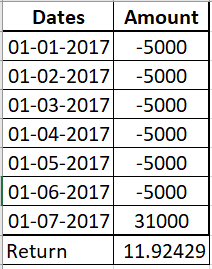sipcalculator

# How to calculate return on mutual fund SIP?

Can someone please explain how are returns calculated on the mutual fund SIPs?

Groww

This is how SIP in mutual funds work -

The first month, you invest 5000 in a mutual fund. According to the NAV (price) of the mutual fund on that day, you receive a certain number of units.

Next month on a pre-selected date (SIP date) you invest another 5000. According to that day's NAV, you get additional units. This goes on, as long as your SIP is running. Over time you accumulate units of the mutual fund.

Any day, the total value of your investment (and redeemable amount) is equal to = total units multiplied by the NAV on that day.

Therefore your returns are calculated as the difference between the selling price and purchase price (multiplied by the units sold).

Pijush Kanti Biswas

An Systematic Investment Plan (SIP) is an option of investing a fixed sum in a mutual fund scheme on a regular basis i.e. predefined regular interval. It is similar to regular saving schemes like a recurring deposit.

For calculating SIP returns, use XIRR. It is function in Excel for calculating internal rate of return or annualized yield for a schedule of cash flows occurring at irregular intervals. It's simple and one doesn't even require the NAV of any date.

For this calculation you need is with example of six-month SIP:

• SIP amount - ₹ 5000
• SIP investment dates- start-01/01/2017, end-01/06/2017
• Redemption date- 01/07/2017
• Maturity amount - ₹ 31000Open an excel sheet and follow these steps:

1. In column A, enter the transaction dates on the left side​.
2. In column B, enter SIP figure of 5000 as a negative figure as it's an outflow.
3. Against the redemption date (Column A), enter redemption amount (Column B) (31000) .
4. In the box below 31000, type in: “ =XIRR (B1:B7, A1:A7)*100 ” and hit enter to get return.

On Groww dashboard, XIRR is showed for each investment (SIP or Lump Sum).

Happy Investing!

Harsh

Returns on any investment is simply = [(Current price - purchase price)/purchase price]. Based on the time period, these returns can be annualized.

In case of SIP, since there are multiple investments (therefore multiple purchase prices) and different time periods for each instalment, calculating returns is a bit tricky. Returns on mutual fund SIP is commonly called XIRR.

Mathematically, XIRR is that single rate of return, which when applied to every instalment (and redemptions if any) would give the current value of the total investment.

How to calculate?

One can use xirr formula in the excel to calculate the returns on SIP. On Groww dashboard, XIRR is showed for each investment (SIP or Lump Sum).

Similar Questions

1st Floor, Proms Complex, SBI

Colony, 1A Koramangala, 560034